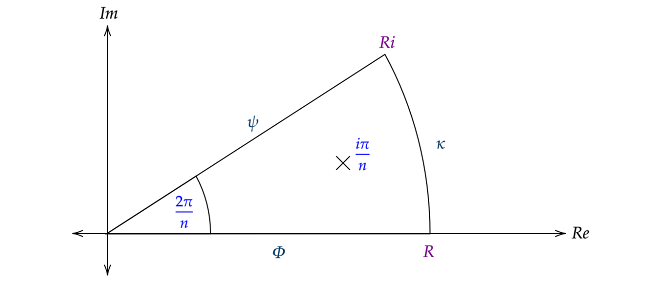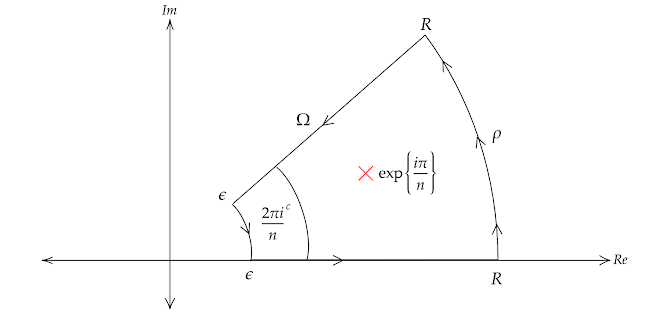## Posts

### On the closed form of the generalized square-sum arctan integralI was recently thinking about the closed-form expression to the $n$th order of the arctan integral in the case of the denominator of the integrated being a sum of squares. In context, the general sum of squares arctan integral is $$\int \dfrac{\mathrm{d}x}{x^2 + a^2} = \dfrac{1}{a}\arctan\left(\dfrac{x}{a}\right) + C$$ $$\int_{0}^{\infty} \dfrac{\mathrm{d}x}{x^2 + a^2} = \dfrac{\pi}{2a}$$ A proof of this employs a basic substitution (factorization combined with $u = \frac{x}{a}$) and the noting of the arctan integral (a result that can be derived via implicit differentiate, with an example given on a previous post). Here, we shall consider the following integral, which we will evaluate using contour integration. $$\int_{\mathbb{R^{+}}} \dfrac{\mathrm{d}x}{x^n + a^n}$$ Factorizing $$\dfrac{1}{a^n} \int_{\mathbb{R^{+}}} \dfrac{\mathrm{d}x}{\left(\frac{x}{a}\right)^n + 1}$$ To simplify the denominator of our integrated, let $u = \frac{x}{a}$, with no change to our bounds, the reciprocal d

### A rediscovery of Stirling's Approximation

I was adamant about trying to find my own way of computing non-integer values of the Gamma function. Extensions of the product definition of the Gamma function wouldn't directly work since those kinds of extensions would yield the same "accuracy" as the extensions of the zeta function to negative integer values. Hence, in doing this, I think I rediscovered a case of Stirling's approximation using a common technique that leverages logarithm laws. Firstly, consider the factorial function, we can see some examples below $$4! = (4)(3)(2)(1) = 24$$ $$7! = (7)(6)(5)(4)(3)(2)(1)$$ $$10! = (10)(9)(8)\cdots(1) = 3628800$$ and onwards, we can generalize this to $$n! = \prod_{\psi=1}^{n} (\psi)$$ Now, a common technique we use to deal with products, which I thought could be applied to this. Consider leveraging logarithm laws to express this finite product in terms of a series, the reason being that we have many more tools to approximate and evaluate series than we do finite prod

### Taking an infinitely small bite### A complex analog to the arctan function

Trigonometric derivatives are fascinating, most specifically inverse trigonometric derivatives. I feel that they are the perfect example to showcase the true power of implicit differentiation. Consider the arctan function. Intuitively, it is difficult to compute any general form for its derivative, however we can do exactly this leveraging a key property of differentiation. Consider the following $$\arctan{x} = y(x)$$ $$x = \tan{[y(x)]}$$ Implicitly differentiating with respect $x$ on both side and applying the chain rule $$1 = y'(x) \cdot \sec^2{y(x)}$$ Noting the following $$y(x) = \arctan{x}$$ $$sec^2 {x} =1+ \tan^2{x}$$ Via substitution, we simplify our equation to  $$1 = y'(x) \cdot (\tan^2{[\arctan{x}}] + 1)$$ $$\dfrac{1}{x^2 + 1} = y'(x)$$ Which is the derivative of the arctan(x) function. Now, with about of curiosity, something quite interesting happens when we leverage this relation. Consider the following $$\int \dfrac{dx}{x^2 + 1} = \arctan{x}$$ Now, let us expa